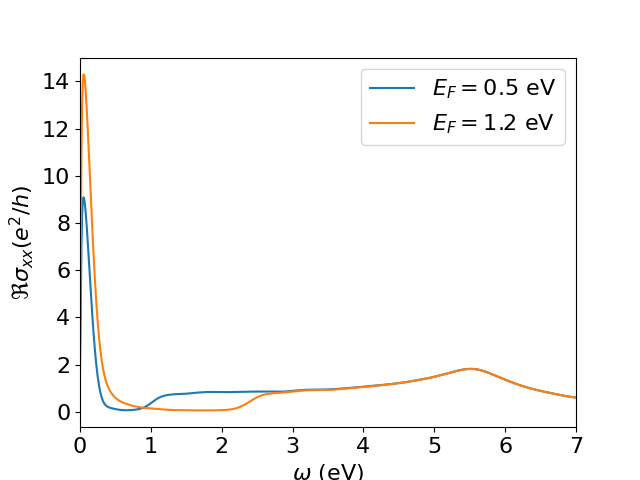# 5. Post-processing

As presented in previous sections, KITEx calculates the Chebyshev moments of a given expansion and stores them in the same HDF file that was generated by the configuration script. These moments are used by the post-processing tool named KITE-tools to reconstruct the requested physical quantities specified in the configuration script, such as the density of states or the optical conductivity.

In a basic setup, the python configuration script specifies the post-processing parameters (e.g., number of energy points), which are exported to the HDF file with other settings. Here, the HDF file containing the Chebyshev moments works as an input, KITE-tools read the post-processing parameters and the Chebyshev moments from the HDF file and uses them to calculate the desired quantities and export them in data files. If a parameter is not specified, a default value is used.

However, many of these quantities depend on a set of physical parameters (such as temperature, chemical potential, etc.) that are not necessary for the calculation of Chebyshev moments. Therefore, the user can modify them and recalculate quantities with KITE-tools without the need of recomputing the Chebyshev moments, the time-consuming part of the calculations. For example: if the user wants to compute the optical conductivity at several Fermi energies, the Chebyshev moments are computed once by KITEx and KITE-tools can be used to obtain the optical conductivities for all Fermi energies.

### Using the post-processing¶

For this purpose, the user has the flexibility to override the parameters from the python script by specifying them in the command line interface. As an example, let us consider the example dos_optcond_gaussian_disorder.py: If we consider the setting

./KITE-tools optcond_gaussian_disorder-output.h5 --CondOpt -F 1.2 -O 0 10 1000 -N optcond1.2.dat


KITE-tools will calculate the optical conductivity with a Fermi energy specified by the option -F 1.2 (in units specified in the configuration file). The option -O sets the frequency interval between 0and 10 (in the same units) and the total number of frequency points (1000). These options will be used to recalculate the optical conductivity even if the original configuration file presents different values. The option -N defines the name of the output file.

We can modify the Fermi energy and produce a different optical conductivities without recalculating the Chebyshev moments:

./KITE-tools optcond_gaussian_disorder-output.h5 --CondOpt -F 0.5 -O 0 10 1000 -N optcond0.5.dat

We can now compare the real component of two different optical conductivitiesComparision between the real components of the optical conductivity of disordered graphene for two Fermi energies.

The same type of strategy can be used to calculate the DC and optical conductivities for different temperatures.

Info

For a more detailed list of possible commands for KITE-tools, look at the API.

Tip

Some functionalities use different postprocessing tools that are not included in KITE-tools.

The single-shot longitudinal conductivity functionality singleshot_conductivity_dc does not store Chebyshev moments in the .h5 file, rather it requests KITEx to directly calculate the dc-conductivity for specified values of the Fermi energy. To extract the calculated DC conductivity from the .h5 file, we can use a python script located in the tools directory: process_single_shot.py. In the same directory, the user can find another script to plot an ARPES spectrum from the output of a spectral function calculation.